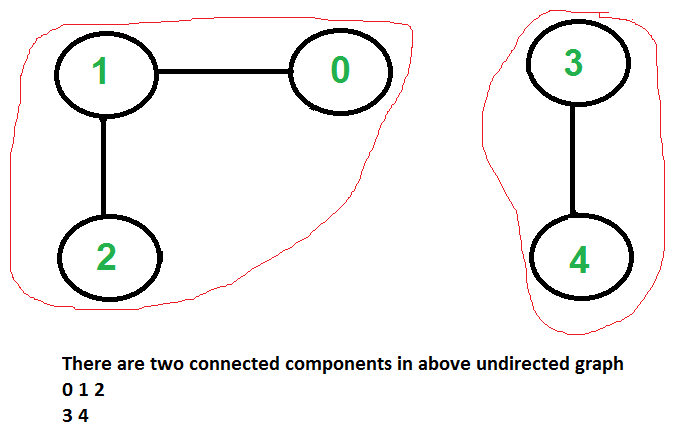Related Articles

# Connected Components in an undirected graph

• Difficulty Level : Medium
• Last Updated : 23 Jun, 2021

Given an undirected graph, print all connected components line by line. For example consider the following graph.We strongly recommend to minimize your browser and try this yourself first.
We have discussed algorithms for finding strongly connected components in directed graphs in following posts.
Kosaraju’s algorithm for strongly connected components
Tarjan’s Algorithm to find Strongly Connected Components
Finding connected components for an undirected graph is an easier task. We simple need to do either BFS or DFS starting from every unvisited vertex, and we get all strongly connected components. Below are steps based on DFS.

```1) Initialize all vertices as not visited.
2) Do following for every vertex 'v'.
(a) If 'v' is not visited before, call DFSUtil(v)
(b) Print new line character

DFSUtil(v)
1) Mark 'v' as visited.
2) Print 'v'
3) Do following for every adjacent 'u' of 'v'.
If 'u' is not visited, then recursively call DFSUtil(u)```

Below is the implementation of above algorithm.

## C++

 `// C++ program to print connected components in``// an undirected graph``#include ``#include ``using` `namespace` `std;` `// Graph class represents a undirected graph``// using adjacency list representation``class` `Graph {``    ``int` `V; ``// No. of vertices` `    ``// Pointer to an array containing adjacency lists``    ``list<``int``>* adj;` `    ``// A function used by DFS``    ``void` `DFSUtil(``int` `v, ``bool` `visited[]);` `public``:``    ``Graph(``int` `V); ``// Constructor``    ``~Graph();``    ``void` `addEdge(``int` `v, ``int` `w);``    ``void` `connectedComponents();``};` `// Method to print connected components in an``// undirected graph``void` `Graph::connectedComponents()``{``    ``// Mark all the vertices as not visited``    ``bool``* visited = ``new` `bool``[V];``    ``for` `(``int` `v = 0; v < V; v++)``        ``visited[v] = ``false``;` `    ``for` `(``int` `v = 0; v < V; v++) {``        ``if` `(visited[v] == ``false``) {``            ``// print all reachable vertices``            ``// from v``            ``DFSUtil(v, visited);` `            ``cout << ``"\n"``;``        ``}``    ``}``    ``delete``[] visited;``}` `void` `Graph::DFSUtil(``int` `v, ``bool` `visited[])``{``    ``// Mark the current node as visited and print it``    ``visited[v] = ``true``;``    ``cout << v << ``" "``;` `    ``// Recur for all the vertices``    ``// adjacent to this vertex``    ``list<``int``>::iterator i;``    ``for` `(i = adj[v].begin(); i != adj[v].end(); ++i)``        ``if` `(!visited[*i])``            ``DFSUtil(*i, visited);``}` `Graph::Graph(``int` `V)``{``    ``this``->V = V;``    ``adj = ``new` `list<``int``>[V];``}` `Graph::~Graph() { ``delete``[] adj; }` `// method to add an undirected edge``void` `Graph::addEdge(``int` `v, ``int` `w)``{``    ``adj[v].push_back(w);``    ``adj[w].push_back(v);``}` `// Driver code``int` `main()``{``    ``// Create a graph given in the above diagram``    ``Graph g(5); ``// 5 vertices numbered from 0 to 4``    ``g.addEdge(1, 0);``    ``g.addEdge(2, 3);``    ``g.addEdge(3, 4);` `    ``cout << ``"Following are connected components \n"``;``    ``g.connectedComponents();` `    ``return` `0;``}`

## Java

 `// Java program to print connected components in``// an undirected graph``import` `java.util.ArrayList;``class` `Graph``{``    ``// A user define class to represent a graph.``    ``// A graph is an array of adjacency lists.``    ``// Size of array will be V (number of vertices``    ``// in graph)``    ``int` `V;``    ``ArrayList > adjListArray;` `    ``// constructor``    ``Graph(``int` `V)``    ``{``        ``this``.V = V;``        ``// define the size of array as``        ``// number of vertices``        ``adjListArray = ``new` `ArrayList<>();` `        ``// Create a new list for each vertex``        ``// such that adjacent nodes can be stored` `        ``for` `(``int` `i = ``0``; i < V; i++) {``            ``adjListArray.add(i, ``new` `ArrayList<>());``        ``}``    ``}` `    ``// Adds an edge to an undirected graph``    ``void` `addEdge(``int` `src, ``int` `dest)``    ``{``        ``// Add an edge from src to dest.``        ``adjListArray.get(src).add(dest);` `        ``// Since graph is undirected, add an edge from dest``        ``// to src also``        ``adjListArray.get(dest).add(src);``    ``}` `    ``void` `DFSUtil(``int` `v, ``boolean``[] visited)``    ``{``        ``// Mark the current node as visited and print it``        ``visited[v] = ``true``;``        ``System.out.print(v + ``" "``);``        ``// Recur for all the vertices``        ``// adjacent to this vertex``        ``for` `(``int` `x : adjListArray.get(v)) {``            ``if` `(!visited[x])``                ``DFSUtil(x, visited);``        ``}``    ``}``    ``void` `connectedComponents()``    ``{``        ``// Mark all the vertices as not visited``        ``boolean``[] visited = ``new` `boolean``[V];``        ``for` `(``int` `v = ``0``; v < V; ++v) {``            ``if` `(!visited[v]) {``                ``// print all reachable vertices``                ``// from v``                ``DFSUtil(v, visited);``                ``System.out.println();``            ``}``        ``}``    ``}` `    ``// Driver code``    ``public` `static` `void` `main(String[] args)``    ``{``        ``// Create a graph given in the above diagram``        ``Graph g = ``new` `Graph(``            ``5``); ``// 5 vertices numbered from 0 to 4` `        ``g.addEdge(``1``, ``0``);``        ``g.addEdge(``2``, ``3``);``        ``g.addEdge(``3``, ``4``);``        ``System.out.println(``            ``"Following are connected components"``);``        ``g.connectedComponents();``    ``}``}`

## Python

 `# Python program to print connected``# components in an undirected graph`  `class` `Graph:` `    ``# init function to declare class variables``    ``def` `__init__(``self``, V):``        ``self``.V ``=` `V``        ``self``.adj ``=` `[[] ``for` `i ``in` `range``(V)]` `    ``def` `DFSUtil(``self``, temp, v, visited):` `        ``# Mark the current vertex as visited``        ``visited[v] ``=` `True` `        ``# Store the vertex to list``        ``temp.append(v)` `        ``# Repeat for all vertices adjacent``        ``# to this vertex v``        ``for` `i ``in` `self``.adj[v]:``            ``if` `visited[i] ``=``=` `False``:` `                ``# Update the list``                ``temp ``=` `self``.DFSUtil(temp, i, visited)``        ``return` `temp` `    ``# method to add an undirected edge``    ``def` `addEdge(``self``, v, w):``        ``self``.adj[v].append(w)``        ``self``.adj[w].append(v)` `    ``# Method to retrieve connected components``    ``# in an undirected graph``    ``def` `connectedComponents(``self``):``        ``visited ``=` `[]``        ``cc ``=` `[]``        ``for` `i ``in` `range``(``self``.V):``            ``visited.append(``False``)``        ``for` `v ``in` `range``(``self``.V):``            ``if` `visited[v] ``=``=` `False``:``                ``temp ``=` `[]``                ``cc.append(``self``.DFSUtil(temp, v, visited))``        ``return` `cc`  `# Driver Code``if` `__name__ ``=``=` `"__main__"``:` `    ``# Create a graph given in the above diagram``    ``# 5 vertices numbered from 0 to 4``    ``g ``=` `Graph(``5``)``    ``g.addEdge(``1``, ``0``)``    ``g.addEdge(``2``, ``3``)``    ``g.addEdge(``3``, ``4``)``    ``cc ``=` `g.connectedComponents()``    ``print``(``"Following are connected components"``)``    ``print``(cc)` `# This code is contributed by Abhishek Valsan`

## C#

 `// C++ program to print connected components in``// an undirected graph``#include ``#include ``using` `namespace` `std;` `// Graph class represents a undirected graph``// using adjacency list representation``class` `Graph``{``    ``int` `V; ``// No. of vertices` `    ``// Pointer to an array containing adjacency lists``    ``list<``int``>* adj;` `    ``// A function used by DFS``    ``void` `DFSUtil(``int` `v, ``bool` `visited[]);` `    ``public` `: Graph(``int` `V); ``// Constructor``    ``~Graph();``    ``void` `addEdge(``int` `v, ``int` `w);``    ``void` `connectedComponents();``};` `// Method to print connected components in an``// undirected graph``void` `Graph::connectedComponents()``{``    ``// Mark all the vertices as not visited``    ``bool``* visited = ``new` `bool``[V];``    ``for` `(``int` `v = 0; v < V; v++)``        ``visited[v] = ``false``;` `    ``for` `(``int` `v = 0; v < V; v++) {``        ``if` `(visited[v] == ``false``) {``            ``// print all reachable vertices``            ``// from v``            ``DFSUtil(v, visited);` `            ``cout << ``"\n"``;``        ``}``    ``}``    ``delete[] visited;``}` `void` `Graph::DFSUtil(``int` `v, ``bool` `visited[])``{``    ``// Mark the current node as visited and print it``    ``visited[v] = ``true``;``    ``cout << v << ``" "``;` `    ``// Recur for all the vertices``    ``// adjacent to this vertex``    ``list<``int``>::iterator i;``    ``for` `(i = adj[v].begin(); i != adj[v].end(); ++i)``        ``if` `(!visited[*i])``            ``DFSUtil(*i, visited);``}` `Graph::Graph(``int` `V)``{``    ``this` `-> V = V;``    ``adj = ``new` `list<``int``>[ V ];``}` `Graph::~Graph() { delete[] adj; }` `// method to add an undirected edge``void` `Graph::addEdge(``int` `v, ``int` `w)``{``    ``adj[v].push_back(w);``    ``adj[w].push_back(v);``}` `// Driver code``int` `main()``{``    ``// Create a graph given in the above diagram``    ``Graph g(5); ``// 5 vertices numbered from 0 to 4``    ``g.addEdge(1, 0);``    ``g.addEdge(2, 3);``    ``g.addEdge(3, 4);` `    ``cout << ``"Following are connected components \n"``;``    ``g.connectedComponents();` `    ``return` `0;``}`

## Javascript

 ``

Output:

```0 1
2 3 4```

Time complexity of above solution is O(V + E) as it does simple DFS for given graph.